# Gas sphere

The gas tank has the shape of a sphere with a diameter of 14 m. How many m3 of gas will fit in it?

V =  1436.8 m3

### Step-by-step explanation: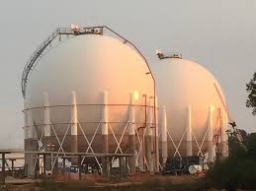Did you find an error or inaccuracy? Feel free to write us. Thank you!Tips to related online calculators
Tip: Our volume units converter will help you with the conversion of volume units.

#### You need to know the following knowledge to solve this word math problem:

We encourage you to watch this tutorial video on this math problem:

## Related math problems and questions:

• The water tankThe water tank has the shape of a sphere with a radius of 2 m. How many liters of water will fit in the tank? How many kilograms of paint do we need to paint the tank, if we paint with 1 kg of paint 10 m2?
• GasholderThe gasholder has spherical shape with a diameter 23 m. How many m3 can hold in?
• Collect rain waterThe garden water tank has a cylindrical shape with a diameter of 80 cm and a height of 12 dm. How many liters of water will fit into the tank?
• The reservoir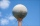The reservoir has the shape of a sphere with a diameter of 7.5 m. How many hectoliters can max fit in a reservoir?
• Tank and waterWe poured a cylindrical tank with 3.5 liters of water. If the tank base diameter is 3 dm, how height is the water level in?
• Fire tank1428 hl of water is filled in a block-shaped fire tank with the edges of the base 12 m and 7 m. Calculate the content of water-wetted areas.
• CannonballsOf the three cannonballs with a diameter of 16 cm, which landed in the castle courtyard during the battle, the castle blacksmith cast balls with a diameter of 10 cm, which fit into the cannons placed on the walls. How many cannonballs did the blacksmith c
• Garden pondConcrete garden pond has bottom shape of a semicircle with a diameter 1.7 m and is 79 cm deep. Daddy wants make it surface. How many liters of water is in pond if watel level is 28 cm?
• How many 9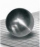How many percent volume remain of a sphere if diameter decrease 3×?
• Three-quarters of its volume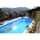The pool has a block shape with a length of 8m, a width of 5.3m and a depth of 1.5m. How many hl of water is in it if it is filled to three-quarters of its volume?
• TentCalculate how many liters of air will fit in the tent that has a shield in the shape of an isosceles right triangle with legs r = 3 m long the height = 1.5 m and a side length d = 5 m.
• TanksThe fire tank has a cuboid shape with a rectangular floor measuring 13.7 m × 9.8 m. Water depth is 2.4 m. Water was pumped from the tank into barrels with a capacity of 2.7 hl. How many barrels were used if the water level in the tank fallen 5 cm? W
• ConvaHow many liters of water fit into the shape of a cylinder with a bottom diameter 20 cm and a height 45 cm?
• The solar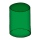The solar heating tank has the shape of a rotating cylinder. When the tank is in a horizontal position, the water in the tank wets 4/5 each tank bases. If we place the tank in a vertical position, the water in the tank will reach up to 1.2 m. Calculate th
• Half-sphere roofThe roof above the castle tower has the shape of a 12.8 m diameter half-sphere. What is this roof's cost if the cost of 1 square meter is 12 euros and 40 cents?
• Water tankA 288 hectoliter of water was poured into the tank with dimensions 12 m and 6 m bottom and 2 m depth. What part of the volume of the tank water occupied? Calculate the surface of tank wetted with water.
• Volume of sphereHow many times does the volume of a sphere increase if its radius increases 2 ×?